NCERT Solutions for Class 6 Maths Chapter 7 Fractions Ex 7.6 are part of NCERT Solutions for Class 6 Maths. Here we have given NCERT Solutions for Class 6 Maths Chapter 7 Fractions Ex 7.6.

 Board CBSE Textbook NCERT Class Class 6 Subject Maths Chapter Chapter 7 Chapter Name Fractions Exercise Ex 7.6 Number of Questions Solved 9 Category NCERT Solutions

## NCERT Solutions for Class 6 Maths Chapter 7 Fractions Ex 7.6

Question 1.
Solve: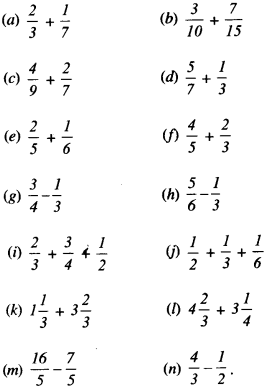Solution :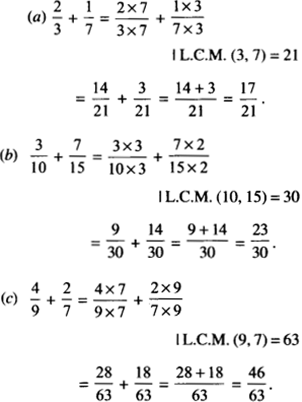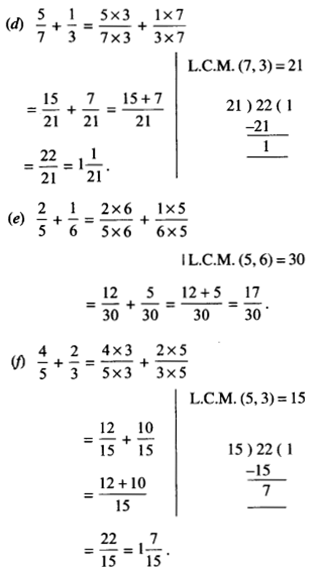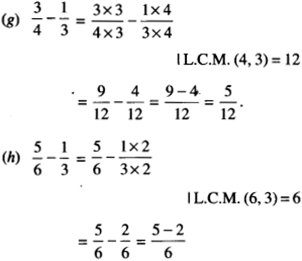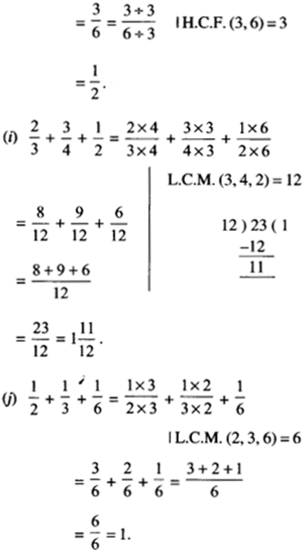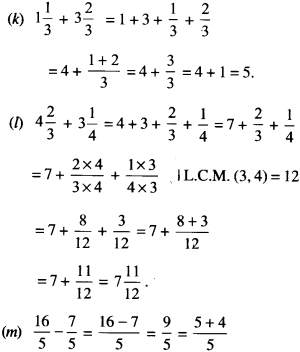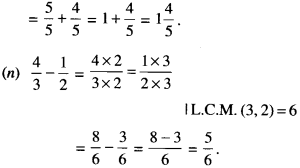Question 2.
Sarita bought $$\frac { 2 }{ 5 }$$ metre of ribbon and Lalita bought $$\frac { 3 }{ 4 }$$ metre of ribbon. What was the total 4 length of the ribbon they bought?
Solution :
Ribbon bought by Sarita = $$\frac { 2 }{ 5 }$$ m
Ribbon bought by Lalita = $$\frac { 3 }{ 4 }$$ m
∴ Total length of the ribbon they bought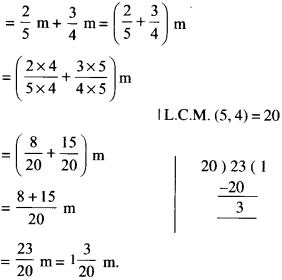Question 3.
Naina was given $$1\frac { 1 }{ 2 }$$ piece of cake and Najma was given $$1\frac { 1 }{ 3 }$$ piece of cake. Find the total amount of care given to both of them.
Solution :
Cake given to naina = $$1\frac { 1 }{ 2 }$$ piece = $$\frac { \left( 1\times 2 \right) +1 }{ 2 }$$ pieceQuestion 4.
Fill in the boxes:Solution :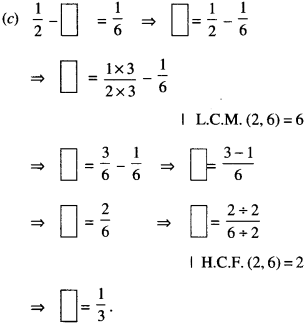Question 5.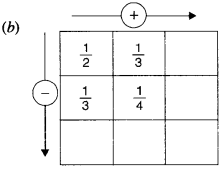Solution :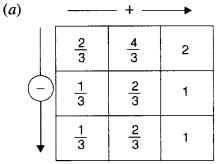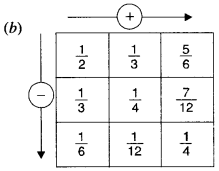Question 6.
A piece of wire $$\frac { 7 }{ 8 }$$ meter long broke into two pieces. One piece was $$\frac { 1 }{ 4 }$$ metre long. How long is the other piece?
Solution :
Length of the original piece of wire = $$\frac { 7 }{ 8 }$$ metre
∴ Length of one piece = $$\frac { 1 }{ 4 }$$ metre
Length of the other piece = $$\frac { 7 }{ 8 }$$ metre – $$\frac { 1 }{ 4 }$$ metre = $$\left( \frac { 7 }{ 8 } -\frac { 1 }{ 4 } \right)$$ metreQuestion 7.
Nandini’s house is ~ km from her school. She walked some distance and then took a bus for km to reach the school. How far did she walk?
Solution :
The distance of Nandini’s house from school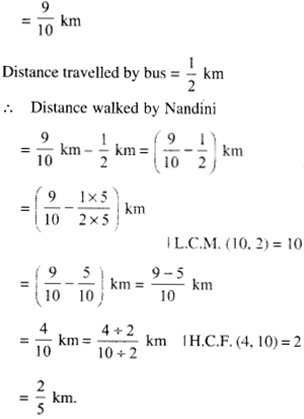Question 8.
Asha and Samuel have bookshelves of the 5 same size. Asha’s shelf is $$\frac { 5 }{ 6 }$$ the full of book and Samuel’s shelf is $$\frac { 2 }{ 5 }$$th full. Whose bookshelf is more full? By what fraction?
Solution :Question 9.
Jaidev takes $$2\frac { 1 }{ 5 }$$ minutes to walk across 5 the school ground. Rahul takes $$\frac { 7 }{ 4 }$$ minutes to do the same. Who takes less time and by what fraction?
Solution :
Time taken by Jaidev to walk across the school ground = $$2\frac { 1 }{ 5 }$$ minutes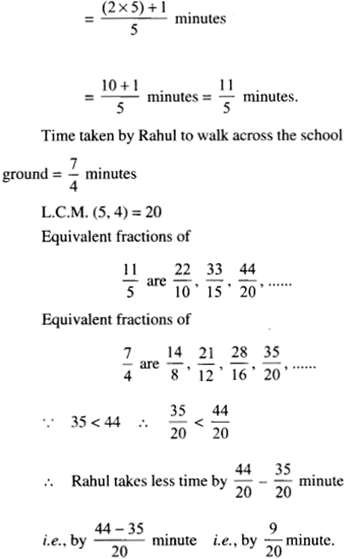We hope the NCERT Solutions for Class 6 Maths Chapter 7 Fractions Ex 7.6 help you. If you have any query regarding NCERT Solutions for Class 6 Maths Chapter 7 Fractions Ex 7.6, drop a comment below and we will get back to you at the earliest.